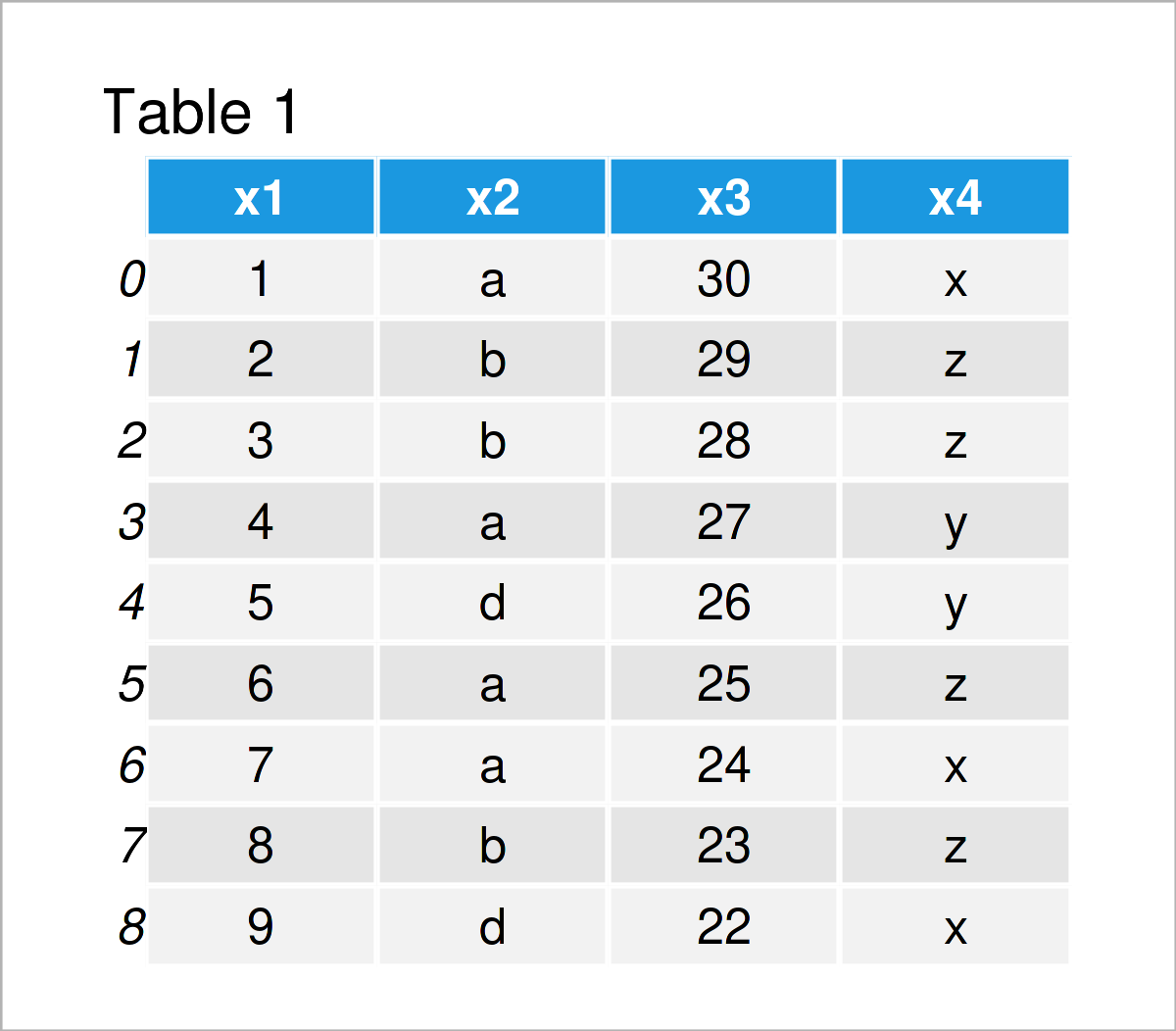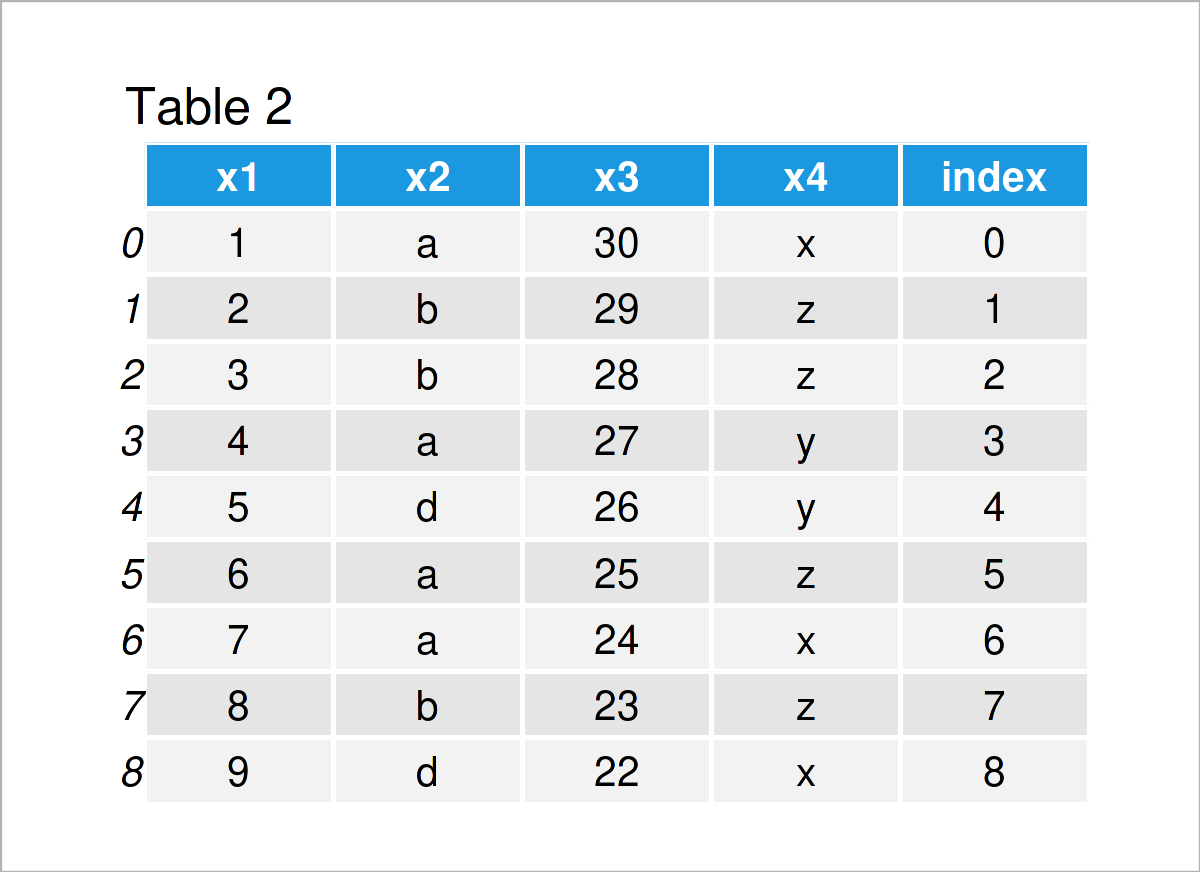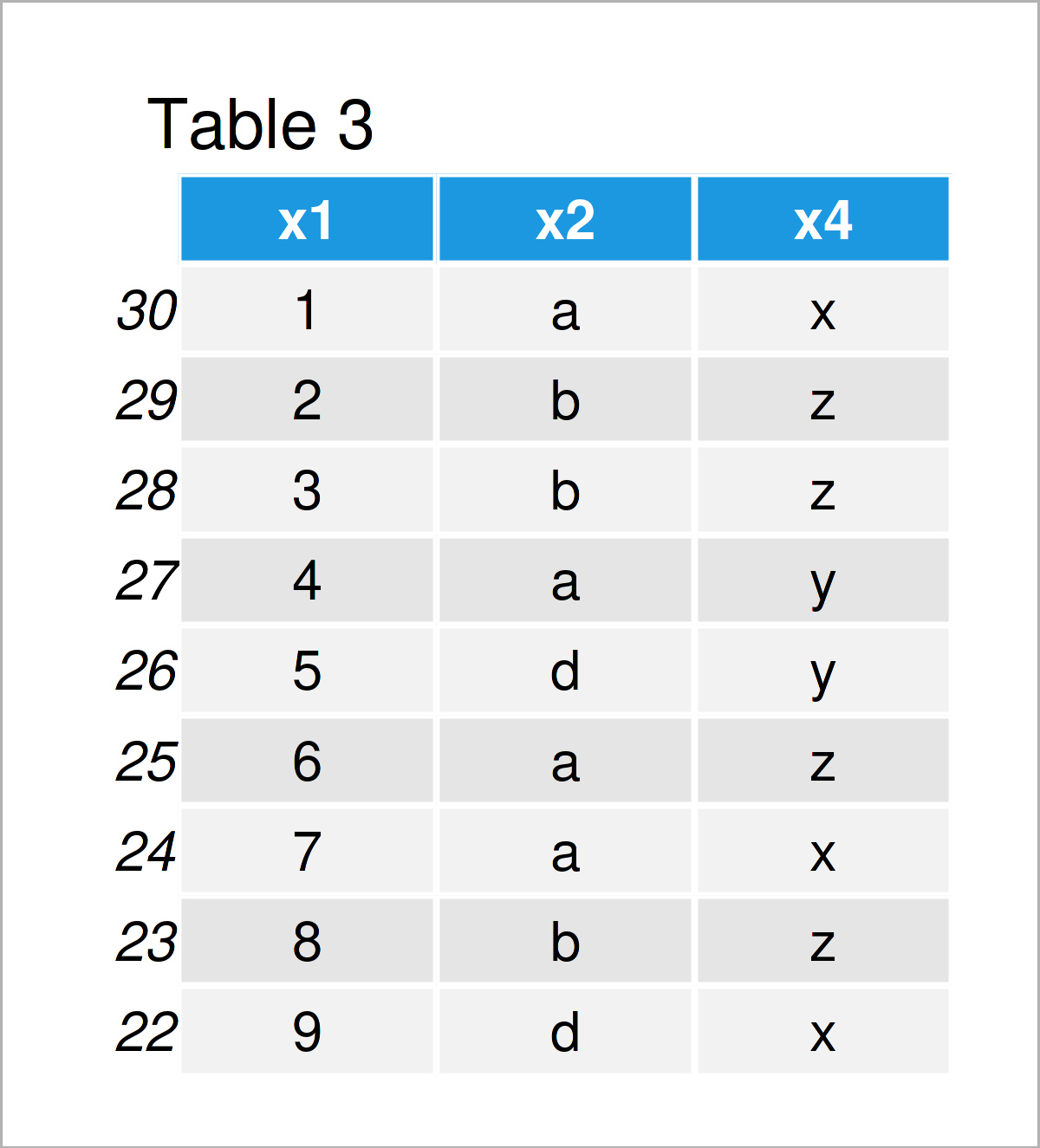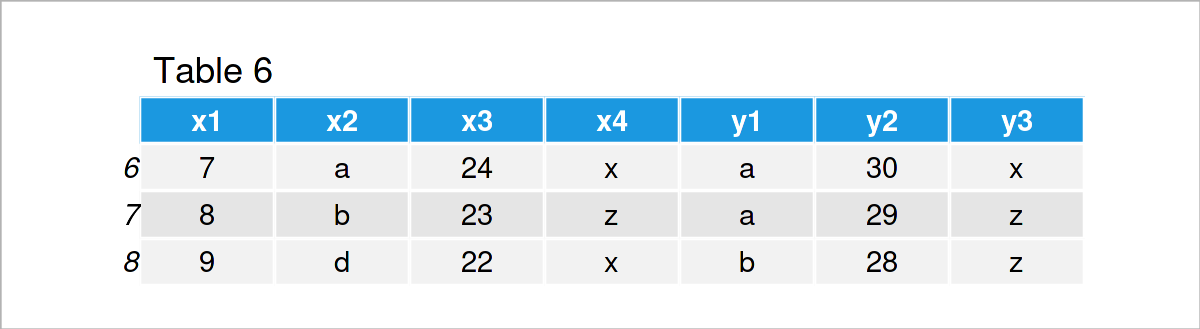# Handling Index of pandas DataFrame in Python (4 Examples)

In this Python tutorial you’ll learn how to manipulate the index of a pandas DataFrame.

Let’s dig in.

## Example Data & pandas Software Library

First, we need to load the pandas library:

`import pandas as pd                                    # Import pandas library`

The following pandas DataFrame will be used as a basement for this Python tutorial:

```data = pd.DataFrame({'x1':range(1, 10),                # Create pandas DataFrame
'x2':['a', 'b', 'b', 'a', 'd', 'a', 'a', 'b', 'd'],
'x3':range(30, 21, - 1),
'x4':['x', 'z', 'z', 'y', 'y', 'z', 'x', 'z', 'x']})
print(data)                                            # Print pandas DataFrame```Table 1 shows that the example data is made of nine rows and four variables.

## Example 1: Convert Index of pandas DataFrame to Column

Example 1 illustrates how to store the index numbers of a pandas DataFrame as an additional column in this DataFrame.

For this task, we can use the index attribute of our pandas DataFrame as shown below:

```data_new1 = data.copy()                                # Duplicate DataFrame
data_new1['index'] = data_new1.index                   # Convert index to column
print(data_new1)                                       # Print updated DataFrame```By executing the previous Python programming code, we have created Table 2, i.e. a new pandas DataFrame containing the index values as an additional column.

## Example 2: Set Column as Index of pandas DataFrame

In this example, I’ll explain how to use a particular variable of a pandas DataFrame as the index (i.e. the opposite of Example 1).

To achieve this, we can use the set_index function as shown below:

```data_new2 = data.set_index('x3')                       # Convert column to index
print(data_new2)                                       # Print updated DataFrame```In Table 3 it is shown that we have created another pandas DataFrame by executing the previous Python programming syntax. The values of the variable x3 have been set as indices for this data set.

## Example 3: Reset Index of pandas DataFrame

Example 3 illustrates how to remove the index numbers of a pandas DataFrame to reset them to a range from 0 to the number of rows of this data set.

We can use the reset_index function to achieve this.

Note that we are using the pandas DataFrame data_new2 that we have created in the previous example. The indices of this DataFrame are not starting at 0.

```data_new3 = data_new2.reset_index()                    # Reindex rows of pandas DataFrame
print(data_new3)                                       # Print updated DataFrame```By executing the previous Python syntax, we have created Table 4, i.e. a pandas DataFrame with reindexed index numbers.

## Example 4: Merge Two pandas DataFrames based on Index

The Python syntax below illustrates how to merge two DataFrames using the index numbers as identifier to match the observations of these two DataFrames.

As a first step for this example, we have to create a second pandas DataFrame:

```data2 = pd.DataFrame({'y1':['a', 'a', 'b', 'c', 'c'],  # Create second pandas DataFrame
'y2':range(30, 25, - 1),
'y3':['x', 'z', 'z', 'x', 'x']},
index = range(6, 11))
print(data2)                                           # Print pandas DataFrame```The output of the previous code is shown in Table 5 – We have created another pandas DataFrame with partly overlapping index numbers with our example data set that we have created at the beginning of this tutorial.

Next, we can use an inner join to merge our two data sets based on their index values:

```data_new4 = pd.merge(data,                             # Join based on index
data2,
left_index = True,
right_index = True)
print(data_new4)                                       # Print updated DataFrame```In Table 6 you can see that we have created a combined version of our two input DataFrames by executing the previous Python code.

## Video & Further Resources

Do you want to learn more about the manipulation of the index of a pandas DataFrame? Then I can recommend watching the following video on my YouTube channel. In the video, I’m explaining the Python programming code of this article.

Please accept YouTube cookies to play this video. By accepting you will be accessing content from YouTube, a service provided by an external third party.If you accept this notice, your choice will be saved and the page will refresh.

Besides the video, you may want to read the other tutorials on this website:

To summarize: In this tutorial you have learned how to handle the indices of a pandas DataFrame in the Python programming language. Please let me know in the comments, if you have any further questions.

Subscribe to the Statistics Globe Newsletter